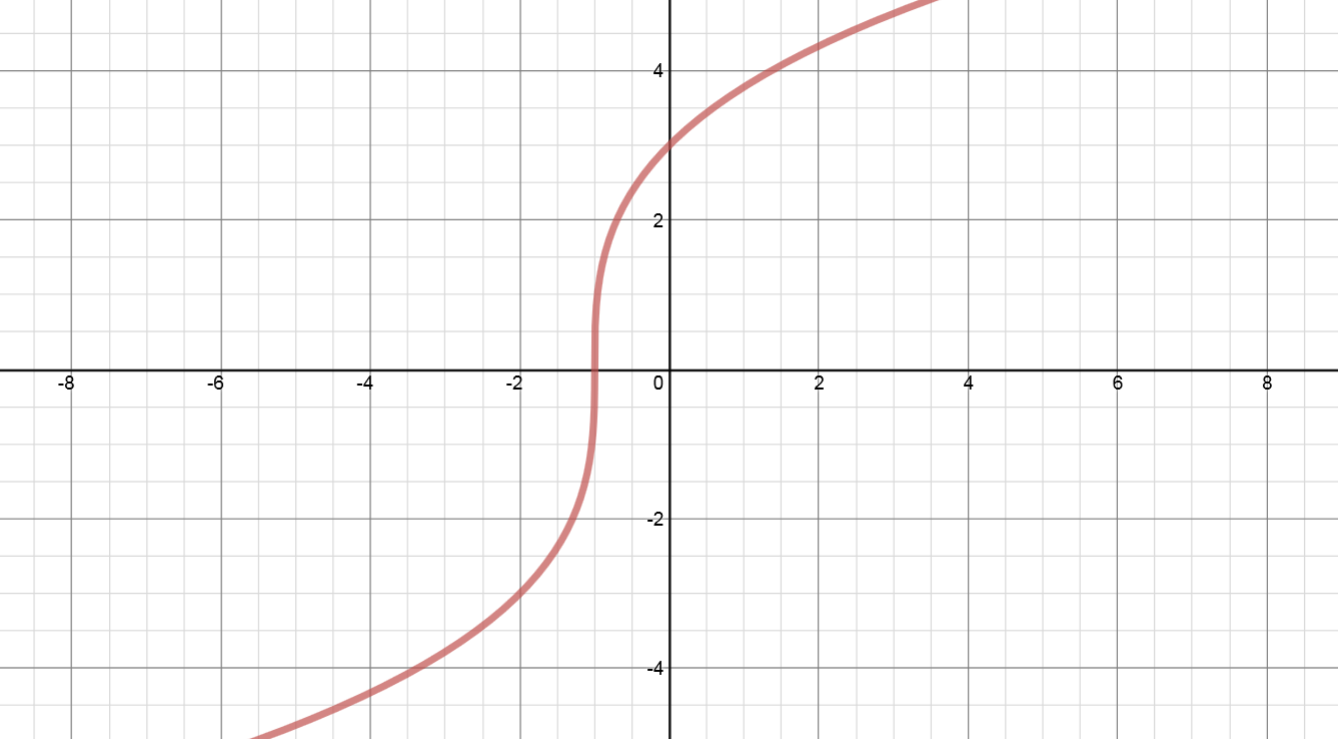Transformations of functions: Horizontal translations

Transformations of functions: Horizontal translations

Horizontal translations refer to movements of a graph of a function horizontally along the x-axis by changing the x values. So, if y = f(x), then y = (x –h) results in a horizontal shift. If h > 0, then the graph shifts h units to the right; while If h < 0, then the graph shifts h units to the left.

Lessons

Compared to $y=f(x)$:
$y=f(x-8)$: shift 8 units to the right
$y=f(x+3)$: shift 3 units to the left
• 1.
a)
Sketch the following functions on the same set of coordinate axes:
$y = {\left( x \right)^2}$      VS.      $y = {\left( {x - 6} \right)^2}$      VS.      $y = {\left( {x + 5} \right)^2}$

b)
Compared to the graph of $y = {x^2}$:
• the graph of $y = {\left( {x - 6} \right)^2}$ is translated "horizontally" ________ units to the ______________.
• the graph of $y = {\left( {x + 5} \right)^2}$ is translated "horizontally" ________ units to the ______________.

• 2.
Horizontal Translations
Given the graph of $y = f\left( x \right)$ as shown, sketch:
a)
$y = f\left( {x-8} \right)$

b)
$y = f\left( {x+3} \right)$

c)
In conclusion:
$\left( x \right) \to \left( {x-8} \right)$: shift __________ to the __________. All x coordinates $\Rightarrow$ ____________________
$\left( x \right) \to \left( {x+3} \right)$: shift __________ to the __________. All x coordinates $\Rightarrow$ ____________________